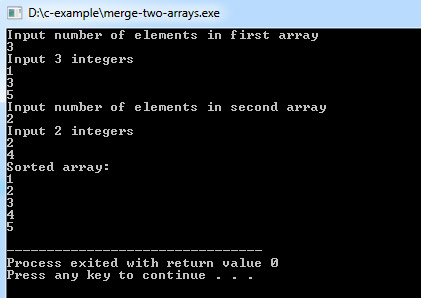## C Programming Examples Tutorial Index

C program to merge two arrays into one array, Arrays are assumed to be sorted in ascending order.
Enter the two short sorted arrays and combine them to obtain a large array.

Program:

``````#include <stdio.h>

void merge(int [], int, int [], int, int []);

int main()
{
int a, b, m, n, c, sorted;

printf("Input number of elements in first array\n");
scanf("%d", &m);

printf("Input %d integers\n", m);
for (c = 0; c < m; c++) {
scanf("%d", &a[c]);
}

printf("Input number of elements in second array\n");
scanf("%d", &n);

printf("Input %d integers\n", n);
for (c = 0; c < n; c++) {
scanf("%d", &b[c]);
}

merge(a, m, b, n, sorted);

printf("Sorted array:\n");

for (c = 0; c < m + n; c++) {
printf("%d\n", sorted[c]);
}
return 0;
}

void merge(int a[], int m, int b[], int n, int sorted[]) {
int i, j, k;
j = k = 0;

for (i = 0; i < m + n;) {
if (j < m && k < n) {

if (a[j] < b[k]) {
sorted[i] = a[j];
j++;
}
else {
sorted[i] = b[k];
k++;
}
i++;
}

else if (j == m) {
for (; i < m + n;) {
sorted[i] = b[k];
k++;
i++;
}
}

else {
for (; i < m + n;) {
sorted[i] = a[j];
j++;
i++;
}
}
}
}``````

Program Output:If arrays are not sorted so you can sort them first and then use the above merge function, another method is to merge them and then sort the array. Two small arrays sorting will take less time than sorting a large array. Merging two sorted arrays is used in merge sort algorithm.